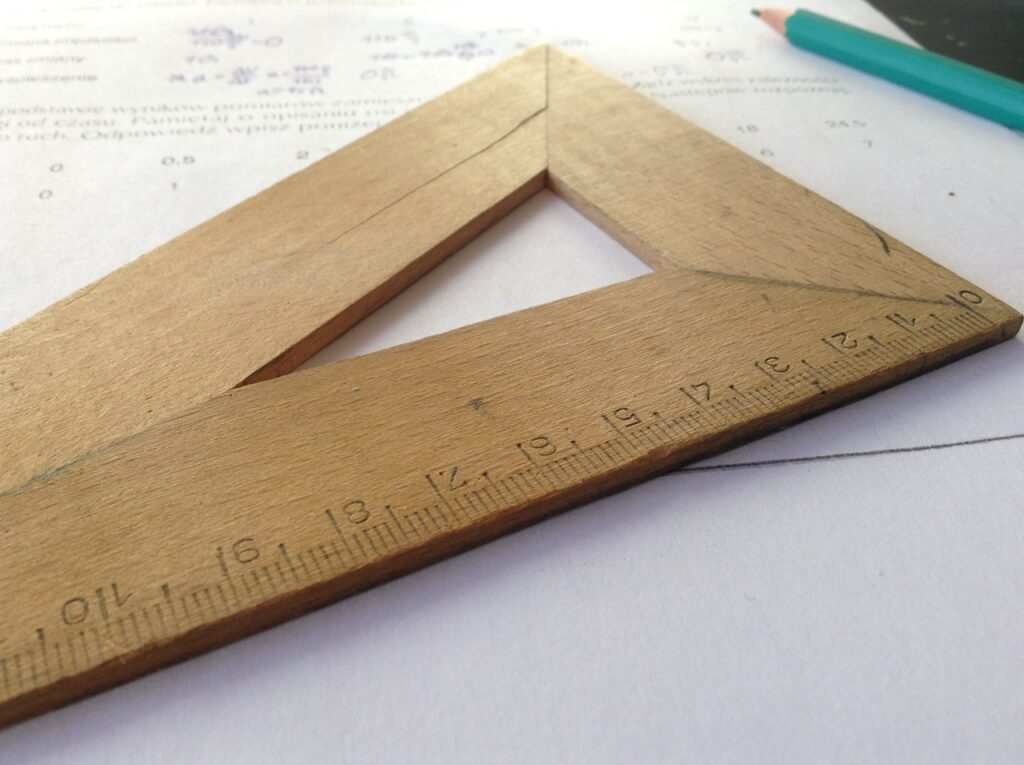## Perimeter and Area Class 6th

Introduction In this section, we shall study the Perimeter and Area for class 6th of plane figures. The Perimeter of the plane figures is the measure related to its Boundary and the Area of the plane figures is the measure related to its region or surface occupied by it. What are Plane Figures? Plane figures …